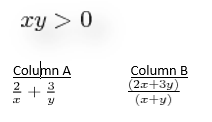+91-22-62437100 / 01 | help@reachivy.com

# GRE Quantitative Comparisons

Quantivative comparisons is a unique question type introduced only in the GRE. In QC questions, you will be given two columns, labeled Quantity A and Quantity B. Additional information may also be provided above the two columns. You have to use the information when evaluating the problem. Quantitative Comparison question tests your ability to analyze information and figure out how to quantities compare to each other.

Instructions: Questions of this type ask you to compare two quantities — Quantity A and Quantity B — and then determine which of four statements describes the comparison.
Directions: Compare Quantity A and Quantity B, using additional information centered above the two quantities if such information is given, and select one of the following four answer choices.
A. Quantity A is greater.
B. Quantity B is greater.
C. The two quantities are equal.
D. The relationship cannot be determined from the information given.

To begin click Next

Question 1:

A, B, and C are 3 consecutive odd integers and A < B < C.

Column X        Column Y
-A - B - 1          -B -C + 3
Question 2:Question 3:

The longest distance between any two points in a right circular cylinder is . The height and the diameter are positive integers where the height exceeds the diameter.

Column A                         Column B
75π                                    The volume of the cylinder
Question 4:

A magazine printer consecutively numbered the pages of a magazine, starting with 1 on the first page, 10 on the tenth page, etc. In numbering the pages, the printer printed a total of 195 digits.

Column A                                         Column B
The number of pages                       100
in the magazine
Question 5:
Rhoda has a Persian rug store, and she makes a profit of $315 on each sale of a rug and each rug costs her$1350.

Column A                                Column B
The profit expressed          The profit expressed
as a percent of the              as a percent of
cost to Rhoda.                     the sale price.
Question 6:

Jessica has to do research for her PhD, but the research report she needs to read costs $10.00, however she can have the x pages of the report she really needs reproduced for$0.20 per page.

Column A                                                         Column  B
The greatest possible value                                 60
of x, if the cost of reproducing the
x pages is less than the cost of
Question 7:

x ≠ 0

Column A
Column B
(7x)4                                   7x4
Question 8:
n, t and z are positive integers and nt = z.

Column A
Column B
n                                z
Question 9:

A cylindrical roller has a base diameter of 4 feet and a length of 8 feet.

Column A                                        Column B

The area covered by the roller                       32,000
after 1,000 revolutions.
Question 10:
A circus has three acts: clowns, stunts, and animals. There are 3 clowns to choose from, 5 stunts, and 4 animals.

Column A                                                    Column B
The maximum number of unique
shows possible if the circus cuts                               60
one act.

You have answered all the questions in the quiz. Press Submit to view your score